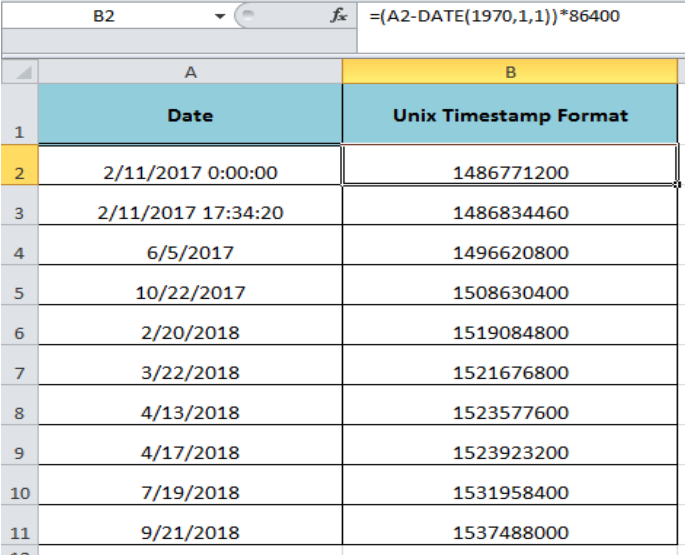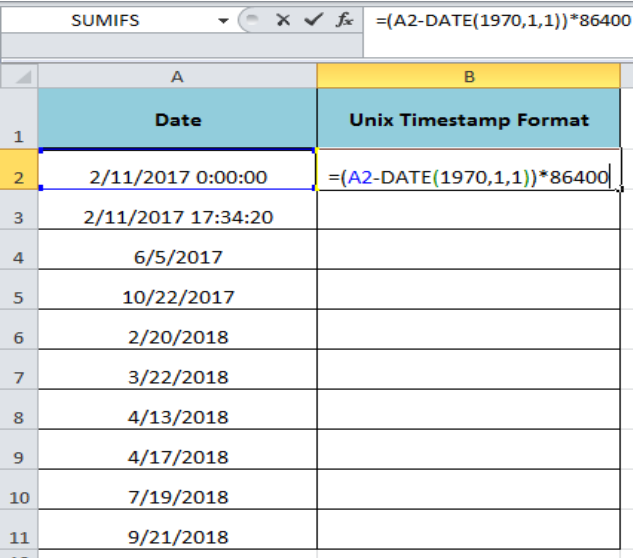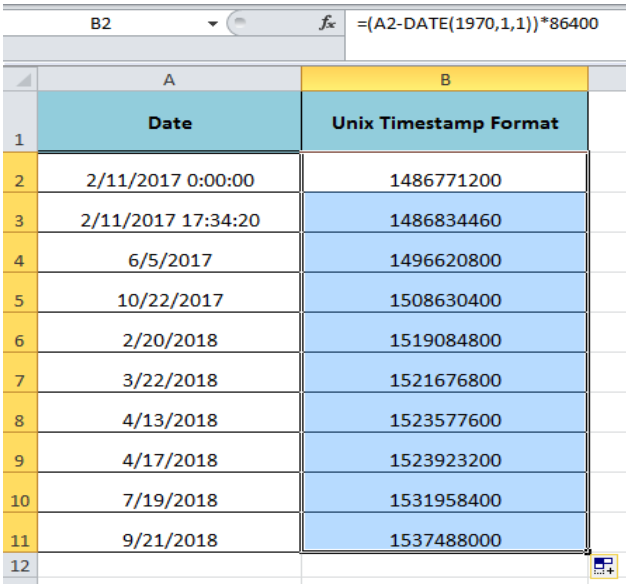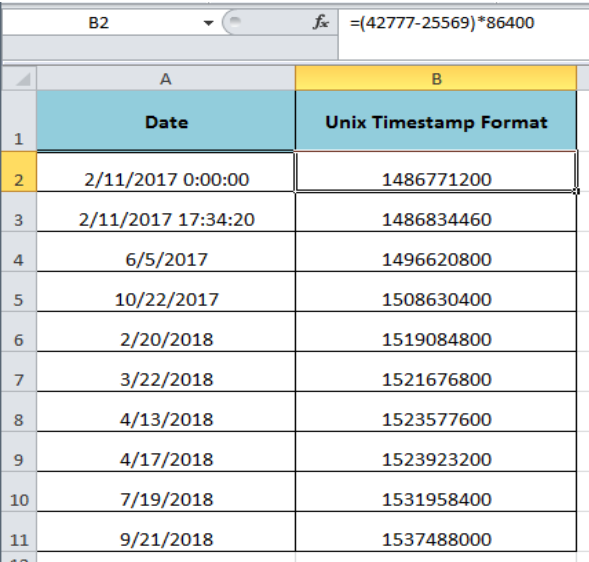Get instant live expert help with Excel or Google Sheets“My Excelchat expert helped me in less than 20 minutes, saving me what would have been 5 hours of work!”

#### Post your problem and you’ll get expert help in seconds.

Your message must be at least 40 characters
Our professional experts are available now. Your privacy is guaranteed.

# How to Convert a Date to Unix Format in Excel

In many file formats or operating systems the Unix time is used which is also referred to as POSIX or Epoch time. The Date and Unix time are different in Excel. Therefore, we need to convert a date into Unix Time in Excel with the help of formula. This article explains the difference between a date and Unix time and how to convert a date to the Unix timestamp format.Figure 1. Converting a Date to Unix Timestamp Format

## Difference Between the Date and Unix Time

The date is in a human-readable form and is used for date handling function with any format type without any conversion containing year, month, day, hour, minute and seconds or just having year, month and day. But the Unix time is a system to describe a point in time and is the number of seconds elapsed since January 1, 1970, 00:00:00 UTC, minus leap seconds. So, the Unix time is basically the total number of seconds between the Epoc time and the given date.

## Converting a Date to Unix Time

With the help of formula, we can convert a date with or without time format to Unix time. As Unix time contains the total number of seconds, so first we need to count the number of days between Excel date and Epoc date and time. Then we multiply the resulting days by 86400 because there are 86400 seconds in a day. In our example, we have a set of dates with and without time formats and by using the following formula we will convert a date to Unix time:

`=(A2-DATE(1970,1,1))*86400`Figure 2. Applying the Formula to Converting a Date to Unix Time

Copy this formula to other cells in column BFigure 3. Copying the Formula

As the date is stored as a serial number in Excel, so the first formula calculates the number of days by subtracting the Epoc date serial number from the given date serial number and then multiply it by 86400 to get the total number of seconds, such as;

`=(42777-25569)*86400`

=`1486771200`Figure 4. Working of the Formula

## Instant Connection to an Expert through our Excelchat Service:

Most of the time, the problem you will need to solve will be more complex than a simple application of a formula or function. If you want to save hours of research and frustration, try our live Excelchat service! Our Excel Experts are available 24/7 to answer any Excel question you may have. We guarantee a connection within 30 seconds and a customized solution within 20 minutes.